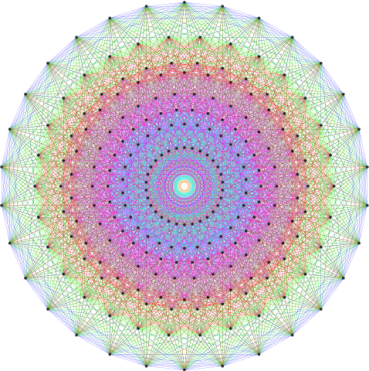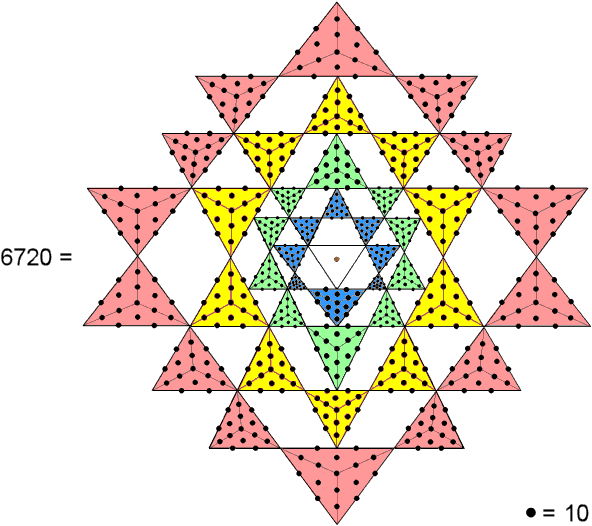Home

 << Previous    1...   14  15    17  18  ...24    Next >>

16. The 2-d Sri Yantra embodies the number of edges of the 421 polytopeThe 421 polytope has 6720 edges. Constructed from Type A triangles, the 2-d Sri Yantra embodies the number 6720 when each extra yod is assigned the number 10.

A Type A triangle contains 19 yods. Of these, three are corners, so that 16 more yods are needed to turn a simple triangle into a Type A triangle. The 42 triangles surrounding the centre of the 2-d Sri Yantra contain (42×16=672) yods other than their 68 corners when they are Type A triangles. In other words, 672 more yods are needed to transform the 42 triangles into Type A triangles. When each yod is weighted with the number 10 (Decad), the 2-d Sri Yantra embodies the number 6720. This is the number of edges in the 421 polytope.

Although traditionally regarded as a triangle, the central, downward-pointing, three-sided figure is not, from a pure mathematical point of view, a triangle because the bindu symbolising the Absolute is assigned to its centre in the case of the 2-d Sri Yantra. For this reason, it is not legitimate to treat this figure as a Type A triangle on the same par as the 42 triangles that surround it.

Of the 672 yods in the 42 Type A triangles, 42 are corners of tetractyses, leaving 630 hexagonal yods. The number 630 is the number value of Seraphim, the Order of Angels assigned to Geburah.

 << Previous    1...   14  15    17  18  ...24    Next >>

Home